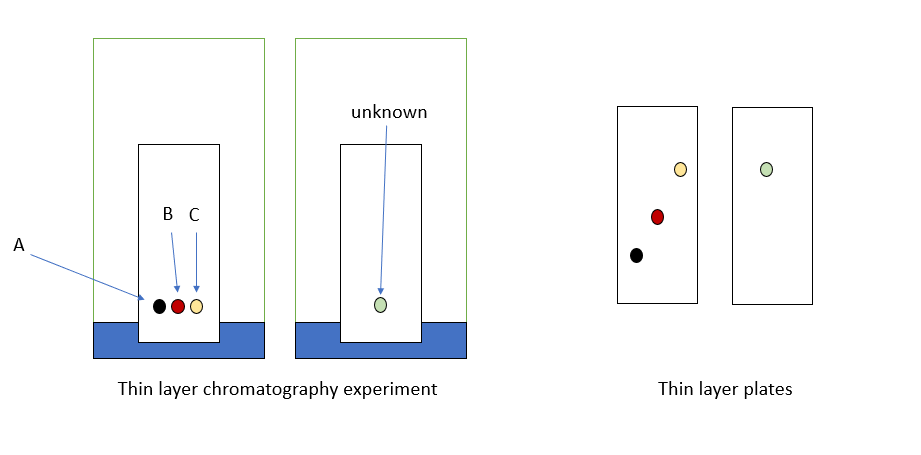# AP Chemistry : Representations of Solutions

## Example Questions

### Example Question #1 : Representations Of Solutions

Which of these solutions can be separated via chromatography?

A homogeneous solution of organic solvents

Ethanol and water

None of the above

Soil and water

B and C

B and C

Explanation:

Chromatography is the physical separation of components of a mixture.  Answers b and c can be separated by chromatography due to their homogeneous nature.  The soil and water example would be separated by filtration.

### Example Question #2 : Representations Of Solutions

Which of the following would most likely form a homogeneous solution?

All would form homogeneous solutions

A and B

NaNO3 and octanol (C8H18O)

NH4Cl and water

Water and benzene (C6H6)

NH4Cl and water

Explanation:

Like dissolves like.  The NH4Cl and water mixture involves an ionic solid and a polar solvent. Examples a and b involve mixing polar/non-polar solvents and ionic and non-polar solvents.

### Example Question #3 : Representations Of Solutions

If 50g of NaCl are dissolved in 5 L of water what is the solute and what is the solvent?

Both are solutes since they are mixed together

NaCl is the solute and H2O is the solvent

They both are solvents because they dissolve into each other

NaCl can not be dissolved in water because NaCl is ionic and water is a polar solvent

NaCl is the solvent and H2O is the solute

NaCl is the solute and H2O is the solvent

Explanation:

The solvent is what you the most off.  In this case water is the solvent and sodium chloride is the solute.

### Example Question #1 : Representations Of Solutions

What would be the best solvent would you choose to dissolve C31H64?

Toluene (C7H8)

Ethanol (C2H5OH)

Acetone (C3H6O)

Water

All of the above

Toluene (C7H8)

Explanation:

C31H64 is a non-polar substance and would dissolve best in a non-polar solvent like Toluene.

### Example Question #1 : Representations Of SolutionsA student investigates a series of compounds in a thin layer chromatography experiment. The student has a sample of three organic compounds labeled A, B, and C as well as an unknown compound.  The student performs the experiment as shown on the left above and gets the results shown on the right.  What organic compound is in the unknown sample?

Sample B

Sample C

None of the above

Sample A + B + C

Sample A